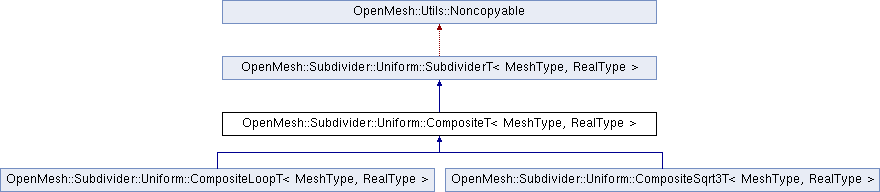Developer Documentation
OpenMesh::Subdivider::Uniform::CompositeT< MeshType, RealType > Class Template Referenceabstract

`#include <libs_required/OpenMesh/src/OpenMesh/Tools/Subdivider/Uniform/Composite/CompositeT.hh>`

Inheritance diagram for OpenMesh::Subdivider::Uniform::CompositeT< MeshType, RealType >:## Classes

struct  Coeff
Abstract base class for coefficient functions. More...

## Public Types

typedef RealType real_t

typedef MeshType mesh_t

typedef SubdividerT< mesh_t, real_t > parent_tPublic Types inherited from OpenMesh::Subdivider::Uniform::SubdividerT< MeshType, RealType >
typedef MeshType mesh_t

typedef RealType real_t

## Public Member Functions

CompositeT (MeshType &_mesh)

virtual const char * name (void) const =0
Return name of subdivision algorithm.Public Member Functions inherited from OpenMesh::Subdivider::Uniform::SubdividerT< MeshType, RealType >
virtual ~SubdividerT ()
Descructor (calls detach())

SubdividerT (void)

SubdividerT (MeshType &_m)

bool operator() (MeshType &_m, size_t _n, const bool _update_points=true)

bool attach (MeshType &_m)

bool operator() (size_t _n, const bool _update_points=true)

void detach (void)

## Protected Types

typedef MeshType::Scalar scalar_t

typedef MeshType::VertexHandle VertexHandle

typedef MeshType::FaceHandle FaceHandle

typedef MeshType::EdgeHandle EdgeHandle

typedef MeshType::HalfedgeHandle HalfedgeHandle

## Protected Member Functions

bool prepare (MeshType &_m)

bool subdivide (MeshType &_m, size_t _n, const bool _update_points=true)
Subdivide mesh `_m` `_n` times.

bool cleanup (MeshType &_m)
Cleanup mesh after usage, e.g. remove added properties.

virtual void apply_rules (void)=0

void commit (MeshType &_m)

void corner_cutting (HalfedgeHandle _heh)
Corner Cutting.

VertexHandle split_edge (HalfedgeHandle _heh)
Split Edge.

Uniform composite subdivision rules
void Tvv3 ()
Split Face, using Vertex information (1-3 split)

void Tvv4 ()
Split Face, using Vertex information (1-4 split)

void Tfv ()
Split Face, using Face Information.

void FF ()
Face to face averaging.

void FFc (Coeff &_coeff)
Weighted face to face averaging.

void FFc (scalar_t _c)
Weighted face to face averaging.

void FV ()
Face to vertex averaging.

void FVc (Coeff &_coeff)
Weighted face to vertex Averaging with flaps.

void FVc (scalar_t _c)
Weighted face to vertex Averaging with flaps.

void FE ()
Face to edge averaging.

void VF ()
Vertex to Face Averaging.

void VFa (Coeff &_coeff)
Vertex to Face Averaging, weighted.

void VFa (scalar_t _alpha)
Vertex to Face Averaging, weighted.

void VV ()
Vertex to vertex averaging.

void VVc (Coeff &_coeff)
Vertex to vertex averaging, weighted.

void VVc (scalar_t _c)
Vertex to vertex averaging, weighted.

void VE ()
VE Step (Vertex to Edge Averaging)

void VdE ()
Vertex to edge averaging, using diamond of edges.

void VdEc (scalar_t _c)
Weighted vertex to edge averaging, using diamond of edges.

void VdEg (Coeff &_coeff)

void VdEg (scalar_t _gamma)

void EF ()
Edge to face averaging.

void EV ()
Edge to vertex averaging.

void EVc (Coeff &_coeff)
Weighted edge to vertex averaging.

void EVc (scalar_t _c)
Weighted edge to vertex averaging.

void EdE ()
Edge to edge averaging w/ flap rule.

void EdEc (scalar_t _c)
Weighted edge to edge averaging w/ flap rule.

## Private Attributes

MeshType * p_mesh_

## Detailed Description

### template<typename MeshType, typename RealType = float> class OpenMesh::Subdivider::Uniform::CompositeT< MeshType, RealType >

This class provides the composite subdivision rules for the uniform case.

To create a subdivider derive from this class and overload the functions name() and apply_rules(). In the latter one call the wanted rules.

For details on the composite scheme refer to

Note
Not all rules are implemented!

Definition at line 94 of file CompositeT.hh.

## Member Function Documentation

template<typename MeshType , typename RealType = float>
 virtual void OpenMesh::Subdivider::Uniform::CompositeT< MeshType, RealType >::apply_rules ( void )
protectedpure virtual

Assemble here the rule sequence, by calling the constructor of the wanted rules.

template<typename MeshType , typename RealType = float>
 void OpenMesh::Subdivider::Uniform::CompositeT< MeshType, RealType >::commit ( MeshType & _m )
inlineprotected

Move vertices to new positions after the rules have been applied to the mesh (called by subdivide()).

Definition at line 150 of file CompositeT.hh.

template<typename MeshType , typename RealType >
 void OpenMesh::Subdivider::Uniform::CompositeT< MeshType, RealType >::VdEg ( Coeff & _coeff )
protected

Weigthed vertex to edge averaging, using diamond of edges for irregular vertices.

Definition at line 778 of file CompositeT.cc.

template<typename MeshType , typename RealType >
 void OpenMesh::Subdivider::Uniform::CompositeT< MeshType, RealType >::VdEg ( scalar_t _gamma )
protected

Weigthed vertex to edge averaging, using diamond of edges for irregular vertices.

Definition at line 719 of file CompositeT.cc.

The documentation for this class was generated from the following files:
• libs_required/OpenMesh/src/OpenMesh/Tools/Subdivider/Uniform/Composite/CompositeT.hh
• libs_required/OpenMesh/src/OpenMesh/Tools/Subdivider/Uniform/Composite/CompositeT.cc